Mathematical and Physical Journal
for High Schools
Issued by the MATFUND Foundation
 Already signed up? New to KöMaL?

# KöMaL Problems in Informatics, September 2015

Show/hide problems of signs:## Problems with sign 'I'

Deadline expired on October 12, 2015.

I. 379. Many settlers in the West knew each other, but they often had no idea about the whereabouts of some other families. A postal service did not yet exist, and only the neighbors met each other. Having no other alternative, they wanted to map their region as follows.

When two neighbors meet, they exchange information. That is, they both let the other know about the people he or she knows about. If this neighbor did not know about someone earlier, then he or she records the new name together with the name of the person sharing this piece of information. Then, if a settler wants to pass on any information to another settler, the sender asks the person to transmit the message who first informed the sender about the recipient's whereabouts.

After sufficiently many encounters, everybody will know about every other settler in the region.

We assume that initially everybody knows their neighbors, but no other families. The file szomszed.txt describes the neighborhood relations, while the file talalkozas.txt contains the encounters in chronological order (who met whom).

Your program should use the standard input to read who wants to send information to whom. Then the program should determine the number of encounters after which the message can be sent, and the route the message travels from the sender to the recipient.

In the example, line breaks in multiple-line inputs have been replaced by the slash character (/); Bemenet'' means input, while Kimenet'' is the corresponding output.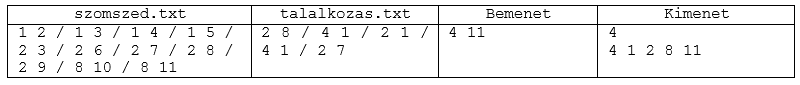The source code of your program, together with a short documentation describing your solution and specifying the developer environment to use for compiling the source, should be submitted in a compressed file i379.zip.

(10 pont)

solution, statistics

I. 380. By using a spreadsheet application it is easy to determine the day of the week for a given date. However, many of these applications cannot interpret dates before 1900 January 1. To handle this situation we can use perpetual calendars that were developed in the pre-computer era. Our perpetual calendar has 3 auxiliary tables. The first auxiliary table (Évek indexszámai'' in the example) assigns an integer (called index) to each year. The second table (Hónapok kulcsszámai'' in the example) yields another number (called key) based on the index and the number of the month. Finally, by using the third table (A kapott számértékhez rendelt nap'' in the example) we find the day of the week from the sum of the key and the number of the day of the month (in the examples, Héfő'' is Monday, and Szombat'' is Saturday). Let us consider 1848 March 15, for example. The index corresponding to 1848 is 45. The key corresponding to 45 and March is 2. Finally, looking up the sum $\displaystyle 2+15$ in the third table we get Wednesday.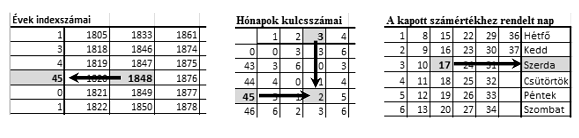In this task you should create a spreadsheet to find the day of the week for a given date. The auxiliary tables are available to you in the (UTF-encoded and tabulator-separated) file tablak.txt.

1. Read the data file tablak.txt to a sheet beginning with cell A1, then save this sheet as oroknaptar in the default application file format.

2. Now you determine the index corresponding to the given year in two steps. First display-in the cells of row 36 and by using a formula-the row number in which the year (=Év'') given in cell C1 appears for each of the columns of the auxiliary table B5:T35. If the year does not appear in a particular column, the corresponding cell in row 36 should contain a 0.

3. As a next step, display-in cell C2 and by using a function-the index of the year in cell C1. The index is found in column A5:A35; its row number is specified by the non-zero element of row 36.

4. Next determine the key (=Kulcs'') and display it in cell E2. The key depends on the index computed above and on the number of the month (=Hónap'') given in cell E1. The key should be determined by using the table A39:M53 and applying a function: columns of this auxiliary table correspond to the months (B39:M39), while rows correspond to the indices (A40:A53).

5. To determine the day (=Nap''), display-in cell G2 and by using a formula-the sum of the key (E2) and the number of the day (G1).

6. In the last step, determine the name of the day. Locate the sum computed in G2 by using a formula in the auxiliary table A57:G63: the name of the day-to be displayed in cell I2-is found in the last column of this table in the row in which the sum appears.

7. Each of the 3 auxiliary tables (A5:T35, A39:M53, A57:G63) should be bordered by thin lines inside and thick lines outside. The content of the 3 cells specifying the date, and that of the cell containing the name of the day should appear in bold with light gray background.

8. By using conditional formatting with bold fonts and dark red color, you should highlight

$\displaystyle a)$ the year in the range B5:T35 that appears in cell C1,

$\displaystyle b)$ the month in the column header B39:M39 that appears in cell E1,

$\displaystyle c)$ the index in the row header A40:A53 that appears in cell C2, and

$\displaystyle d)$ the integer in the range B40:M53 that appears in cell G2 (as Számérték'').

Sample: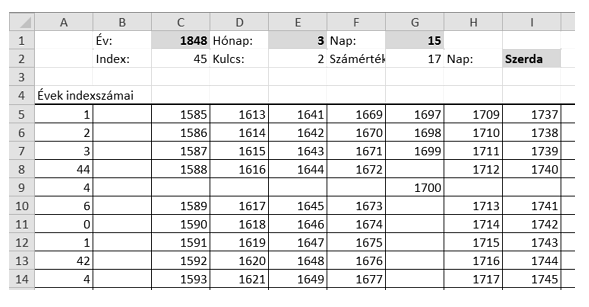You should submit your sheet and a short documentation in a compressed file i380.zip.

(10 pont)

solution, statistics

I. 381. In this exercise you are asked to highlight the new features of the HTML5 and CSS3 languages by creating a webpage that also uses these languages.

The starting page index.html should have a header, a footer, and a middle part with the actual content. The header should contain the appropriate logos on the left, then the title HTML5-CSS3 New Features''. The content part should contain 300px-wide boxes of the same height, each illustrating a new feature. There should be altogether 8 boxes, each containing an image of the same size and a sentence, together with a link to a webpage with more detailed explanation. Among these boxes there should be one where the link points to your page kedvenc.html. This kedvenc.html page should elaborate on one topic with a working example. The structure of kedvenc.html should be similar to that of your main page; one box in the content part should contain the description of your favorite new topic. The footer should be used as an imprint, containing your data (name, school year, town) and the logos for validating HTML5 and CSS3.

The look and formatting of the two webpages should be controlled by a single file forma.css. Your page should be displayed properly on screens with different sizes-depending on the screen width you may want to rearrange your page. For example, the logos in the header and footer should appear only on medium or larger screens, but text characters should always be completely visible. On the other hand, the boxes on your main page should be distributed evenly; on larger screens 4 boxes should appear in one row, then gradually less, finally, one box per row on the smallest screens. As for the colors, your pages should be based on different shades of gray, other colors should only be used in the images.

A compressed file i381.zip should be submitted containing the folder with your webpage.

(10 pont)

solution, statistics## Problems with sign 'I/S'

Deadline expired on October 12, 2015.

I/S. 1. A low-voltage circuit contains a thin metal plate, and a machine removes certain disks from this plate. Your task in this exercise is to decide whether the circuit will still be closed after the removal of the disks, that is, whether there is a path between points $\displaystyle A$ and $\displaystyle B$.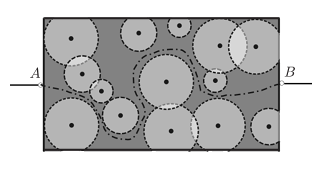The metal plate is an $\displaystyle N\times M$ rectangle ($\displaystyle 10\le N,M\le 1000$, with $\displaystyle N$ and $\displaystyle M$ specifying its width and height, respectively). The machine cuts out $\displaystyle K$ disks ($\displaystyle 0\le K\le 100$), and it is possible for the disks to intersect one another. The center of the $\displaystyle i^\mathrm{th}$ disk is given by ($\displaystyle x_{i}$, $\displaystyle y_{i}$) with some integer coordinates within the rectangle, while its radius is $\displaystyle r_i$ ($\displaystyle 0 < r_{i} \le \min(N,M)$ with $\displaystyle r_i$ being some integer).

Points $\displaystyle A$ and $\displaystyle B$ are connected to the rectangle through the complete vertical segments $\displaystyle x=0$ and $\displaystyle x=N$, respectively. When a disk is removed, its interior and boundary are all deleted, so if two disks touch each other, for example, then their point of contact is also removed from the plate.

Your program should read the values of $\displaystyle N$, $\displaystyle M$ and $\displaystyle K$ from the first line of the standard input, then the coordinates of the disk centers and the radii (positive integers) from the next $\displaystyle K$ lines. The first and only line of the standard output should be either ''Conducts'' or ''Does not conduct'', depending on whether or not electricity can pass through the circuit after the removal of the disks.

In the example, Példa bemenet'' is a sample input, while Példa kimenet'' is the corresponding output.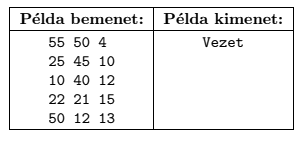Scoring and bounds. You can get 1 point for a brief and proper documentation clearly describing your solution. Nine further points can be obtained provided that your program solves any valid input within 1 second of running time.

The source code of your program with a short documentation of your solution, and also describing which developer environment to use for compiling the source, should be submitted in a compressed file is1.zip.

(10 pont)

solution, statistics## Problems with sign 'S'

Deadline expired on October 12, 2015.

S. 100. We are given $\displaystyle N$ squares ($\displaystyle 1\le N\le 300\;000$) with sides parallel to the coordinate axes. The size of each square is $\displaystyle K\times K$ ($\displaystyle 1\le K\le 1\;000\;000$), and the center of each square is also given by a pair $\displaystyle (x; y)$ ($\displaystyle -1\;000\;000\le x, y\le 1\;000\;000$). Some squares do not overlap, but sometimes one or more pairs of squares overlap with an intersection having positive area.

Your program should read $\displaystyle N$ and $\displaystyle K$ from the first line of the standard input, then the $\displaystyle x_i$, $\displaystyle y_i$ coordinates from the following $\displaystyle N$ lines. The first and only line of the standard output should be either 0 if no two squares overlap, or -1 if there are more than one overlapping pairs, or the area of the intersection if there is exactly one pair of overlapping squares.

In the example, Példa bemenet'' is a sample input, while Példa kimenet'' is the corresponding output. Explanation: squares 1 and 3 overlap.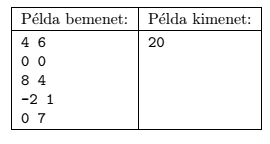Scoring and bounds. You can get 1 point for a brief and proper documentation clearly describing your solution. Nine further points can be obtained provided that your program solves any valid input within 1 second of running time.

The source code of your program with a short documentation-also describing which developer environment to use for compiling the source-should be submitted in a compressed file s100.zip.

(10 pont)

solution, statistics

\$Var(Body)